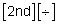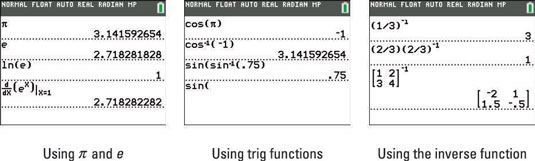##### TI-84 Plus CE Graphing Calculator For Dummies, 3rd EditionStarting with the fifth row of the TI-84 Plus calculator, you find the functions commonly used on a scientific calculator. Here’s what they are and how you use them:

• π and e

The transcendental numbers π and e are respectively located in the fifth and sixth rows of the last column of the keyboard. To enter π in the calculator, press [2nd][^]; to enter e, press• The trigonometric and inverse trigonometric functions

The trigonometric and inverse trigonometric functions are located in the fifth row of the keyboard. These functions require that the argument of the function be enclosed in parentheses. To remind you of this, the calculator provides the first parenthesis for you.

• The inverse function

The inverse function is located in the fifth row of the left column on the calculator. To enter the multiplicative inverse of a number, enter the number and press [x–1]. When dealing with matrices, using the [x–1] key will calculate the inverse of the matrix. The third screen shows these operations.

If you want to evaluate an arithmetic expression and you need a function other than those just listed, you’ll most likely find that function in the Math menu.

You can impress your friends at parties by pointing out that TI-84 Plus uses 3.1415926535898 for π in calculations.